Get instant live expert help with Excel or Google Sheets“My Excelchat expert helped me in less than 20 minutes, saving me what would have been 5 hours of work!”

#### Post your problem and you’ll get expert help in seconds.

Your message must be at least 40 characters
Our professional experts are available now. Your privacy is guaranteed.

# How to use formula bar in Excel – Excelchat

We use the formula bar to display and modify formulas. The formula bar is a toolbar that appears at the top of our worksheet, below the Ribbon in our workbook. The formulas bar, sometimes called fx bar, displays text or number data in active or current cells, formulas in an active cell or range of cells in place of a selected data series in an Excel chart.

## Editing formulas, charts, and data

By simply clicking on any cell in our range of data, we can find the formula contained in that active cell in the formula bar.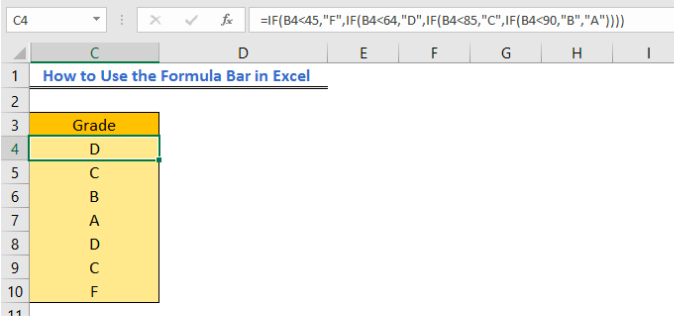Figure 1 – Show Excel Formula bar

## How to Hide or Show the Formula bar

1. To view the formula bar in excel, we will click on the View tab in the ribbon and check the formula option.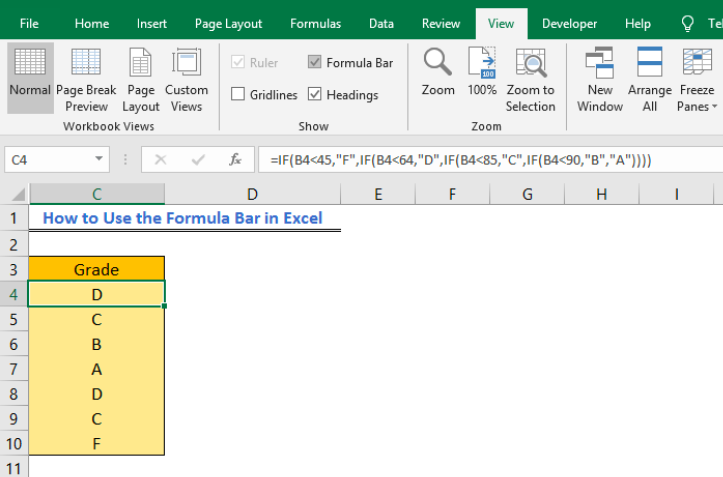Figure 2 – How to show formula bar

2. To hide the formula bar in excel, we will click on the View tab in the ribbon and uncheck the formula option. The formula bar will no longer be visible at the top of the worksheet.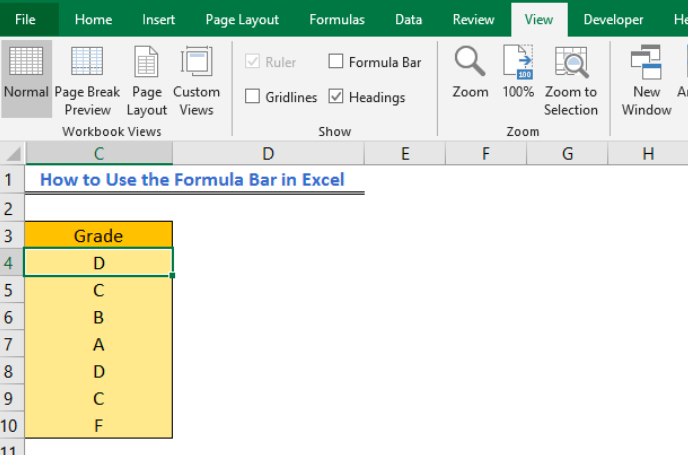Figure 3 – Hide formula bar

## Expanding the formula bar in Excel

If we are working with complex formulas or long data entries, we can expand the formula bar in excel. To do this;

• We will hover the mouse pointer around the bottom of the formula bar until it becomes a two-headed arrow

• We will press and hold down the left mouse button and pull down to expand the formula bar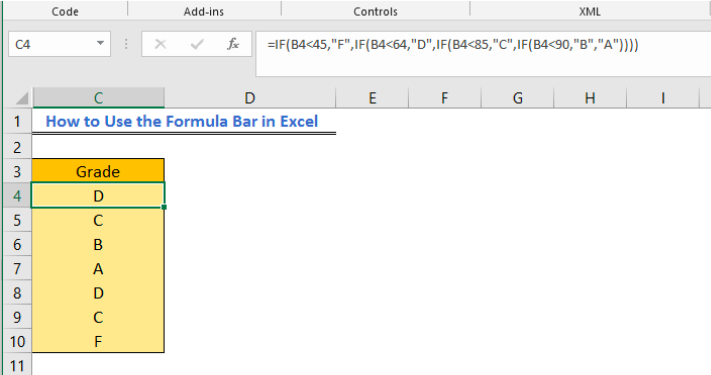Figure 4 – Show formula bar in expanded format

• Now, we can wrap formula bar on multiple lines by clicking to place insertion point and then press Alt + Enter on the keyboard. Our formula from the break will move to the next line in the formula bar.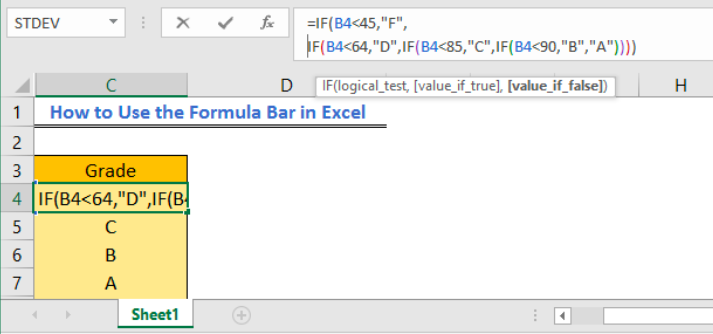Figure 5 – Wrap formula across multiple lines

• Alternatively, we can use the keyboard shortcut Ctrl +Shift +U to expand formula bar.

## Hide Formula bar using Worksheet protection

We can prevent the formula bar from showing by using the Excel worksheet protection. First, we will hide the cells containing formulas and then apply for worksheet protection

• We will select the range of cells containing the formulas to hide
• In the Home Tab, we will click on the Format option in the drop-down menu
• Here, we will click on Format Cells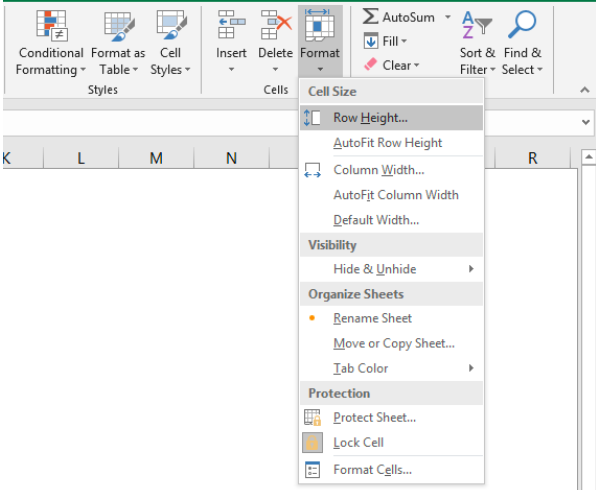Figure 6 – Hide Formulas with password protection

• In the Format Cells dialog box, we will select the Protection tab and mark the Hidden checkbox. We will press OK to apply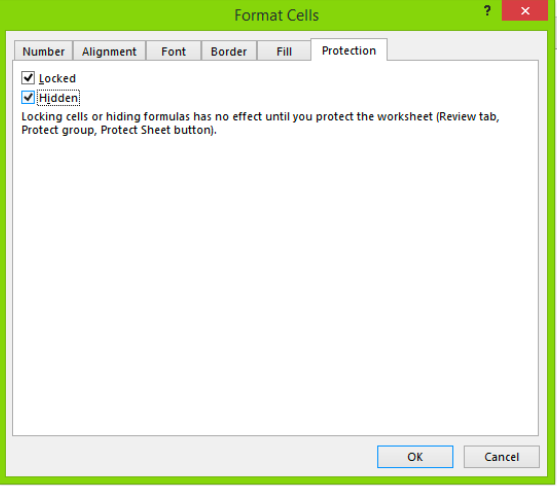Figure 7 – Hide formulas in formula bar in Excel

• Next, in the Home Tab, we will select the Format option to open the drop-down menu.
• We will tap the Protect Sheet button at the bottom of the list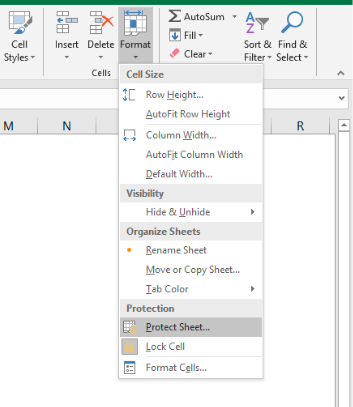Figure 8 – Hide formulas in Excel Formula bar

• In the Protect Sheet dialog box, we will mark or unmark our desired options.
• We will click OK to apply changes and close the dialog box.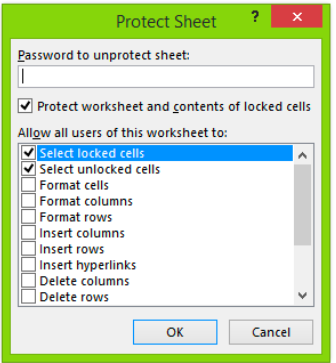Figure 9 – Protect worksheet dialog box

Whenever we click into any of the cells, we will not be able to see the formula in the formula bar as shown below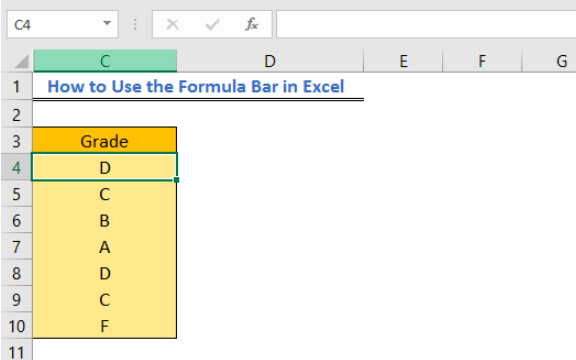Figure 10 – How to hide formulas in Excel formula bar

## Instant Connection to an Expert through our Excelchat Service

Most of the time, the problem you will need to solve will be more complex than a simple application of a formula or function. If you want to save hours of research and frustration, try our live Excelchat service! Our Excel Experts are available 24/7 to answer any Excel question you may have. We guarantee a connection within 30 seconds and a customized solution within 20 minutes.